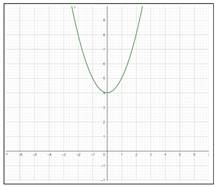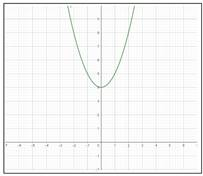# To draw : a graph by using a graphing calculator.### Precalculus: Mathematics for Calcu...

6th Edition
Stewart + 5 others
Publisher: Cengage Learning
ISBN: 9780840068071### Precalculus: Mathematics for Calcu...

6th Edition
Stewart + 5 others
Publisher: Cengage Learning
ISBN: 9780840068071

#### Solutions

Chapter 2.3, Problem 14E

a.

To determine

## To draw: a graph by using a graphing calculator.

Expert Solution

### Explanation of Solution

Given information: consider the provided function,

f(x)=x2+4

Graph:

Step 1: Open Graphing Calculator from the start menu.

Step 2: Click on the input box and enter the function provided f(x)=x2+4

Step 3: Press Enter.

Hence, the graph so obtained is,b.

To determine

### the domain and the range from the graph

Expert Solution

the domain from the graph is [,] and the range from the graph is [4,] .

### Explanation of Solution

Given information: consider the graph obtained in part a.,Calculation:

The domain of the function f is all the x-values of the points on the graph, and the range is all the corresponding y -values.

From the graph, the function cuts the y -axis at y = 4 and covers the graph till , therefore, the range is [4,] . The function covers the graph from x= to x= , therefore, the domain is [,]

Hence, the domain from the graph is [,] and the range from the graph is [4,] .

### Have a homework question?

Subscribe to bartleby learn! Ask subject matter experts 30 homework questions each month. Plus, you’ll have access to millions of step-by-step textbook answers!